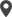Enable Dark Mode!By: Mehjabin Farsana P

# How to add computed fields to a model in Odoo 13?

## Technical Odoo 13

In Odoo, sometimes we may require special types of fields having values calculated from other fields. We may require calculated values from the same records or in the related records. One of the common examples for the usage of such fields is calculating the total amount that is calculated by multiplying the unit price of the product with quantity. So instead of reading a database stored value, we can also calculate the values for the fields by a function in Odoo. In Odoo models, we can achieve this by the use of computed fields.
This blog explains all about how to add computed fields to a model in Odoo.

A computed field is declared just like any other regular field in Odoo along with the additional argument compute. The value is the name of the function as a string or a function. To see how computed fields work let’s go through the following example:
Suppose Cybrosys needs to add the total weight of products in the sale order. So we can define two computed fields here, one for calculating the weight of the product in the orderline and the second one for calculating the total weight by adding all the line weights.
Adding the line_weight computed field in Orderline
Let’s define the line_weight field as follows

`line_weight=fields.Float(string='Line Weight (Kg)',compute='_compute_line_weight')`

Here we have used the argument compute and the _compute_line_weight is the name of the function used to calculate the line weight. After defining the computed field as given above we can define the computed function as follows.
Defining the computed function

`    def _compute_line_weight(self):        for line in self:            line_weight = 0            if line.product_id and line.product_id.weight:                line_weight += (line.product_id.weight                                * line.product_uom_qty / line.product_uom.factor)            line.line_weight = line_weight`

In this function, it calculates the line weight by multiplying the product weight with the orderline quantity and appends the weight to the line_weight field.
Now let’s define another computed field to calculate the total weight of the order as follows:

`   total_weight=fields.Float(string='Total Weight (Kg)', compute='_compute_total_weight')`
Defining the '_compute_total_weight' function
Here also we have given the argument compute and so now let’s define the '_compute_total_weight' function as given below:

`    def _compute_total_weight(self):        for rec in self:            total_weight = 0            for line in rec.order_line:                if line.product_id:                    total_weight += line.line_weight or 0.0            rec.total_weight = total_weight`

This function calculates the total weight of products by adding each line weight and updating the field total_weight.
So this is how computed fields in Odoo works.
The definition of computed fields in Odoo is exactly the same as any other regular fields in Odoo. The only exception is the attribute compute which is used to specify the name of the function used for its computation. Computed fields are dynamically calculated at the runtime. They are not writable or searchable unless you specifically add that support by yourself.
The computation function is dynamically calculated at the runtime. But the ORM uses caching to avoid inefficiently recalculating the value every time its value is accessed. So it needs to know what other fields are depending on. We can use the @depends decorator to defect when its cached value should be invalidated and recalculated.
The optional store = True flag makes the field stored in the database. In this case, after being computed the field values are stored in the database. So they can be retrieved just like any other regular fields instead of being recomputed at runtime. The ORM will know at what time these stored values need to be recomputed and updated.
The compute_sudo = True flag is used in those cases where the computations needed to be done with elevated privileges. This can be the case when the computation needs to use data that may not be accessible to the end-user.
It is also possible to make searchable a non-stored computed field by setting the search attribute to the function name to use. Write support can be added by implementing the inverse function. It uses the value assigned to the computed field to update the origin fields.
It is essential that the compute function always sets a value on the computed field. Otherwise, an error will be raised. So this is how the computed fields in Odoo work.

If you need any assistance in odoo, we are online, please chat with us.

### Recent Posts

#### How to Manage Inventory Operation Types in Odoo 16Calicut

Cybrosys Technologies Pvt. Ltd.
Neospace, Kinfra Techno Park
Kakkancherry, Calicut
Kerala, India - 673635London

Cybrosys Limited
Alpha House,
100 Borough High Street, London,
SE1 1LB, United KingdomKochi

Cybrosys Technologies Pvt. Ltd.
1st Floor, Thapasya Building,
Kochi, India - 682030.Bangalore

Cybrosys Techno Solutions
The Estate, 8th Floor,